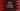# Python program to count total digits in a string in four ways## Python program to count total digits in a string:

In this post, we will learn how to count the total number of digits in a string in Python. This can be done in different ways and we will use different approaches to solve it.

For example, for the string hello123, we have 3 numbers and for the string hello world, we have 0 numbers. Our program will take one string as the input from the user and print the total numbers as the output.

### Method 1: By using a loop:

We can use a loop to iterate over the characters of a string and for each digit found, we can increment one counter variable by 1 to calculate the total number of digits.

Below is the complete program:

``````given_str = input('Enter a string: ')

count = 0

for ch in given_str:
if ch.isdigit():
count += 1

print(count)``````

Here,

• We are taking one string as input from the user and that string is stored in the variable given_str.
• count variable is initialized as 0. This variable is used to hold the total number of digits in the string.
• We are using one for loop, that iterates through the characters of the string one by one. For each character, it checks if that character is a digit or not by using the isdigit() method. If it is a digit, it increments the value of count by 1.
• At the end of the program, it is printing the value of count, i.e. total digits found in the user given string.

If you run this program, it will print output as like below:

``````Enter a string: hello123 world
3``````

### Method 2: By using sum():

We can also do it in one line as like below:

``````given_str = input('Enter a string: ')

count = sum(c.isdigit() for c in given_str)

print(count)``````

Here, sum gives the total digits found in the string. If you run this program, it will print similar output.

### Method 3: By using map():

We can also use map() and isdigit() to find the total digits in a string in Python. Below is the complete program:

``````given_str = input('Enter a string: ')

count = sum(map(str.isdigit, given_str))

print(count)``````

It will give similar output.

### Method 4: By using regular expression:

We can use regular expression or regex to match all numbers in a string and finding the length of that string will give us total count of digits.

``````import re

given_str = input('Enter a string: ')

count = len(re.sub('[^0-9]', '', given_str))

print(count)``````

We are using the re module. It will give similar result.